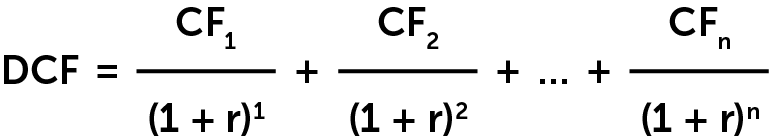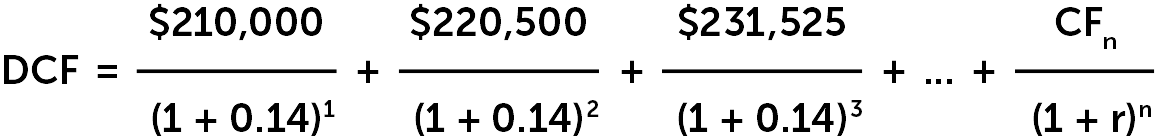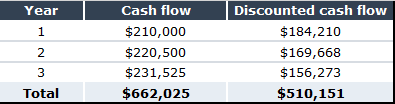# Stock Analysis: How does Discounted Cash Flow work?Jul 20, 2022

How do you know how much an investment is worth? Conducting a discounted cash flow (DCF) analysis is the best way to arrive at an educated guess, whether you’re looking at the cost for a specific project, purchasing shares of a publicly traded company or investing in a private business.

DCF is an intrinsic valuation method like discounted cash flow analysis, which looks at the value of an investment based on its projected future cash flows.

This helps investors gauge a company’s current market value and then assess if that company is under- or overvalued.

Here’s a quick overview of how discounted cash flow analysis works.

What is discounted cash flow analysis?

DCF analysis is an intrinsic valuation method used to estimate the value of an investment based on its forecasted cash flows. It establishes a rate of return or discount rate by looking at dividends, earnings, operating cash flow or free cash flow that is then used to establish the value of the business outside of other market considerations.

In other words: It looks to answer the question, “How much money will I get from this investment over a period of time and how does that compare to the amount I could make from other investments?”

It does this by adjusting for the time value of money—which assumes a dollar invested today is worth more than a dollar invested tomorrow because it’s generating interest over that period.

How do you conduct DCF analysis?

To conduct a DCF analysis, assumptions must be made about a variety of factors, including a company’s forecasted sales growth and profit margins (its cash flow) as well as the rate of interest on the initial investment in the business, the cost of capital and potential risks to the company’s underlying value (aka discounted rate). The more insight into a company’s financials you have, the simpler it is to do.

With so many variables though, it’s easy to see why pricing a deal can be difficult and why most investors and transaction advisors choose to use multiple types of valuation models to inform their decision-making along with DCF analysis. An accurate answer helps inform how much an investment is currently worth—and which deals are worth walking away from.

What are the main inputs of a DCF model?

Discounted cash flow models typically consider three values:

• DCF—Discounted cash flow, which is the sum of all future discounted cash flows that an investment is expected to produce
• CF—Cash flow for a given year
• r—Discount rate, or the target rate of return on the investment expressed in decimal form

Here’s the basic discounted cash flow formula for a simplified analysis:Keep in mind, there are a wide range of formulas used for DCF analysis outside of this simplified one, depending on what type of investment is being analyzed and what financial information is available for it. This formula is simply meant to highlight the general reasoning used in the process.

What is an example of calculating DCF analysis?

Let’s say you’re looking at buying a 10% stake in a private company. It has an established business model that’s profitable and its revenue is growing at a consistent rate of 5% per year. Last year, it produced \$2 million in cash flow, so a 10% stake would’ve likely given you \$200,000 had you purchased it last year.

Here’s a simplified explanation of how DCF analysis could help you determine how much you should reasonably pay for that 10% stake:

This year, the business would give you \$210,000, assuming the company’s established 5% YoY revenue growth. Next year, \$220,500, and so on, assuming the company’s growth rate stays consistent.

Let’s also assume your target compound rate of return is 14%—that is to say, the rate of return you know you can likely achieve on other investments. This means you wouldn’t want to purchase the stake in the business unless you knew you could achieve at least that rate of return; otherwise, you’re better off investing your money elsewhere. Because of this, 14% becomes the discount rate (r) you apply to all future cash flows for the prospective investment.The numerators in the equation above represent the expected annual cash flows, assuming a 5% YoY growth rate. Meanwhile, the denominators convert those cash flows into their present value since they’re divided by your target 14% annual compound interest. The DCF is the sum of all future cash flows and is the most you should pay for the stake in the company if you want to realize at least 14% annualized returns over whatever time period you choose.

For the sake of simplicity, let’s say you’re only looking out three years for this investment. The table below illustrates how in this example, even as the expected cash flows of the company keep growing, the discounted cash flows will shrink over time. That’s because the discount rate is higher than the growth rate—meaning you’d make more money on your investment elsewhere, provided you were certain you could reach your target rate of return through other means.In this scenario, even though you’d hypothetically receive \$231,525 in cash flow in year three of your investment, that would only be worth \$156,273 to you today. Reason: If you invested that same amount today and realized a 14% YoY return on it through another investment, you would have turned it into \$231,525 in that same time period. And because the discount rate (14%) is higher than the growth rate of the company’s cash flow (5%), the discounted versions of those future cash flows will continue to decrease in value each year until they reach zero.

Assuming your target YoY rate of return is 14% for this investment and your exit window is three years out, \$510,151—the total DCF for that time period—is the most you should pay for the 10% stake at this time.

What are the advantages of DCF analysis?

• DCF allows for objective valuations independent of market factors. Since it calculates value apart from subjective market sentiment, it is considered a more objective valuation method than other alternatives. The market can often misprice companies, and the DCF is unaffected by temporary market distortions.
• DCF considers the long-term value of a potential investment. DCF assesses the potential earnings of an investment over the long-term, considering the time value of money and allowing investors to estimate how long it will take them to see a certain level of return.
• DCF is an extremely detailed approach to valuations. DCF uses specific numbers and metrics that are derived based off assumptions about a business, including cash flow projections and discount rates. If these assumptions are accurate, it can provide a good picture of a company or investment’s value.

What are the disadvantages of DCF analysis?

• DCF analysis depends on accurate estimates. DCF models rely heavily on the assumptions previously mentioned, and if these estimations are inaccurate, it can have a significant impact on the investor. For example, if an investor estimates future cash flows to be too high, it can result in an investment that won’t pay off in the future, hurting profits. Conversely, assuming cash flows to be too low can result in missed opportunities.
• A DCF model requires significant data. Creating a DCF model requires a significant amount of financial information that can be difficult and timely to obtain and analyze.